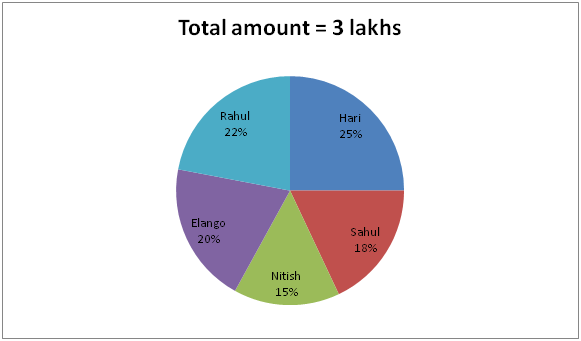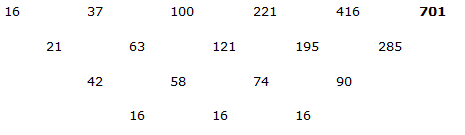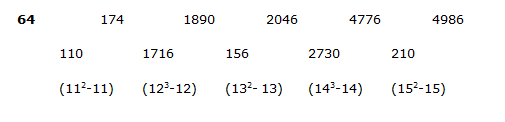# IBPS PO Mains 2018 – Quantitative Aptitude Questions Day-4

Dear Readers, Bank Exam Race for the Year 2018 is already started, To enrich your preparation here we have providing new series of Practice Questions on Quantitative Aptitude – Section. Candidates those who are preparing for IBPS PO Mains 2018 Exams can practice these questions daily and make your preparation effective.

[WpProQuiz 3974]

Directions (1-5): Study the following graph carefully and answer the given questions.

The pie chart shows the percentage distribution of the amount they had in their hand.1) Hari and Elango started a business by investing 60% and 50% of their amount respectively. After one year, Hari increased his capital by 20% and Elango decreased his capital by 30%. At the end of two years Hari received Rs.66000, then find the total profit?

a) Rs.2 lakhs

b) Rs.1.5 lakhs

c) Rs.1 lakh

d) Rs.1.6 lakhs

e) None of these

2) Nitish started a business by investing two – third of the amount. After 3 months, Rahul joined with him by investing Rs. (x+5000) and Nitish received Rs.24000 from the total profit of Rs.39000 at the end of a year, then what percentage of amount invested by Rahul out of the total amount he had? (Approx)

a) 24%

b) 32%

c) 48%

d) 38%

e) 56%

3) Sahul, Nitish and Rahul started a business by investing 45%, 30% and 54% of their amount respectively. Find the profit ratio of Sahul, Nitish and Rahul at the end of a year?

a) 41: 24: 61

b) 45: 25: 66

c) 48: 32: 61

d) 61: 52: 63

e) None of these

4) Hari and Sahul invested the whole amount in scheme A and B which offers Simple and Compound interest respectively. If Scheme A offers 15% and scheme B offers 10%, then find the interest received by Hari and Rahul at the end of two years?

a) Rs. 54680

b) Rs. 37810

c) Rs. 33840

d) Rs. 57850

e) Rs. 39980

5) The whole amount invested by Elango is what percent less than the whole amount invested by Hari?

a) 20%

b) 25%

c) 30%

d) 36%

e) 48%

Directions (6-10): Find the wrong number in the following series.

6) 16, 37, 100, 221, 416, 700

a) 416

b) 221

c) 100

d) 37

e) 700

7) 44, 87.5, 349, 2092, 16730

a) 16730

b) 2092

c) 349

d) 87.5

e) 44

8) 64, 320, 80, 400, 100, 450

a) 400

b) 450

c) 100

d) 80

e) 320

9) 60, 174, 1890, 2046, 4776, 4986

a) 174

b) 1890

c) 2046

d) 60

e) 4776

10) 1258, 630, 316, 159, 80

a) 80

b) 158.5

c) 316

d) 630

e) 1258

Direction (1-5) :

Amount invested by Hari = 300000 * 25/100 * 60/100 = 45000

Amount invested by Elango = 300000 * 20/100 * 50/100 = 30000

Profit ratio of Hari and Elango

= (45000*1+45000 * 120/100 * 1): (30000*1+30000*70/100*1)

= (45000+54000): (30000+21000)

= 99000: 51000

= 33: 17

Hari’s share = 33/50 of total share = Rs.66000

Total share = 66000/33 * 50 = Rs.1 lakh

Amount invested by Nitish = 300000*15/100 * 2/3 = 30000

Amount invested by Rahul = (x+5000)

Profit ratio of Nitish and Rahul = 24000: (39000-24000)

= 24000: 15000 = 8: 5

According to the question,

(30000 * 12): [(x+5000)*9] = 8/5

30000*12/(x+5000) *9 =8/5

15000/(x+5000) = 3/5

75000 = 3x + 15000

3x = 60000 => x= 20000

Total amount invested by Rahul = 20000 + 5000 = 25000

Required percentage = [25000/(300000*22/100)]*100

= (25000/66000)*100

= 38 % (Approx.)

Profit ratio of Sahul, Nitish and Rahul

= [300000 * (18/100)*(45/100)] : [300000 * (15/100)*(30/100)] : [300000* (22/100)*(54/100)]

= (18*45) : (15*30) : (22*54)

= (3*45) : (15*5) : (22*9)

= 45 : 25 : 66

Interest earned by Hari in SI = [(300000 * 25/100)*15*2/100)

= 75000*30/100 = Rs. 22500

Amount received by Sahul in CI = (300000*18/100) (1+10/100)2

= 54000 * (11/10 * 11/10)

= Rs. 65340

Interest received by Sahul in CI = 65340 – 54000

= Rs. 11340

Total interest = 225000 + 11340 = Rs. 33840

Required percentage = [(25-20)/25]*100 = (5/25)*100

= 20%

Direction (6-10) :44 * 2 – 0.5 = 87.5

87.5 * 4 – 1 = 349

349 * 6 – 2 = 2092

2092 *8 – 4 = 16732 (not 16730)

64 * 5 = 320

320 /4 = 80

80 * 5 = 400

400 /4 = 100

100 * 5 =500 (not 450)1258/2 + 1 = 630

630/2 + 1 =316

316/2 +1 = 159

159/2 + 1 =80.5 (not 80)

Daily Practice Test Schedule | Good Luck

 Topic Daily Publishing Time Daily News Papers & Editorials 8.00 AM Current Affairs Quiz 9.00 AM Current Affairs Quiz (Hindi) 9.30 AM IBPS PO Mains – Reasoning 10.00 AM IBPS PO Mains – Quantitative Aptitude 11.00 PM Vocabulary (Based on The Hindu) 12.00 PM IBPS PO Mains – English Language 1.00 PM IBPS PO/Clerk – GK 3.00 PM Daily Current Affairs Updates 5.00 PM IBPS Clerk Prelims – Reasoning 6.00 PM IBPS Clerk Prelims – Reasoning (Hindi) 6.30 PM IBPS Clerk Prelims – Quantitative Aptitude 7.00 PM IBPS Clerk Prelims – Quantitative Aptitude (Hindi) 7.30 PM IBPS Clerk Prelims – English Language 8.00 PM